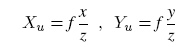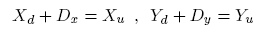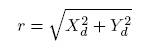\$darkmodeVCG Library## Viewing and manipulation

In this section, all that concerns the definition of a view and the manipulation of a mesh will be explained.

## Shot and camera

This section presents the structure of the shot and camera of the library. After an overview of the camera model used, all the components of the shot class are listed and described. Then, a set of examples of the most important operations (projection and un-projection) and of the interactions between shots and between a shot and the trackball are presented. Finally, simple examples of a shot are visually shown, in order to help with the implementations of eventual wrapped to and from other shot-camera formats.

The camera model

In generale, the camera parameters can be divided in two groups:

• Extrinsic (or external) parameters: these are the parameters associated to the position in the space of the camera.
• Intrinsic (or internal) parameters: these values are related to the peculiar characteristics of the camera, like the focal length (the zoom) or the distortion introduced by the lenses.

If these group of values are put in a proper camera model, it is possible to transform any point in the space in the corresponding point on the image plane of the camera (and viceversa).An example scheme of a perspective camera model

In fact, given a simple perspective camera model like the one shown in figure, extrinsic parameters can be used to transform a point from its world coordinates {xw,yw,zw} to the camera 3D coordinate system {x,y,z}):In this case the extrinsic parameters are a 3 X 3 rotation matrix R and a translation vector T, which define the orientation and position of the camera. In order to transform the 3D camera coordinate in 2D image plane coordinates (Xu,Yu) it's necessary to know the measure of the distance between the point of view and the image plane (OO1 in figure): this value, indicated with f, is usually known as the focal length. The relation between the camera and image coordinates of the point can be expressed as follows:Another aspect of the structure of a camera that can be characterized is the distortion introduced by the lenses: if we suppose that the distortion is radial (performed along the radial direction respect to the center of distortion) we can calculate the undistorted image coordinateswhereandIn conclusion, a quite accurate model of a camera can be described by:

• A 3x3 rotation matrix and a translation vector for extrinsic parameters
• The values of focal, center of distortion and one or more distortion coefficients for intrinsic parameters

While this set of parameters provides everything to transform any 3D point in its corresponding point in the image plane, this could be not enough in peculiar applications. If an accurate estimation of the real position of the camera respect to the object is needed, some more data about the camera model are needed: in particular, the sensor physical size together with the resolution in pixel of the acquired image. If these a-priori data are known, a unique set of camera parameters is associated to any shot.

The VCG Shot

The implementation of a Shot in the VCG library can be found in vcg\math\shot.h

The shot is composed by two elements:

• the Extrinsics parameters, which are stored in the class Shot (in the type ReferenceFrame) that contains viewpoint and view direction. The Extrinsics parameters are kept as a rotation matrix "rot" and a translation vector "tra" NOTE: the translation matrix "tra" corresponds to -viewpoint while the rotation matrix "rot" corresponds to the axis of the reference frame by row, i.e.
rot[0 1 2] == X axis
rot[0 1 2] == Y axis
rot[0 1 2] == Z axis
It follows that the matrix made with the upper left 3x3 equal to rot and the 4th column equal to tra and (0,0,0,1) in the bottom row transform a point from world coordinates to the reference frame of the shot.
• the Instrinsics parameters, which are stored as a Camera type (check vcg/math/camera) and that determines how a point in the frame of the camera is projected in the 2D projection plane. This information was kept indendent of the extrinsic parameters because more than one shot can share the same intrinsic parameters set.

The attributes of a Camera, which is define in vcg\math\shot.h, are:

//------ camera intrinsics
ScalarType FocalMm;
Point2<int> ViewportPx;
Point2< S> PixelSizeMm;
Point2< S> CenterPx;
Point2< S> DistorCenterPx;
S k;
//------------------------

While the extrinsic parameters usually change between the shots, some (sometimes all) of the intrinsics are strongly related to the camera model used. In particular, some values are usually known before camera calibration: viewportPx and CenterPx. Moreover, if an accurate calibration is needed, it is necessary to fill the PixelSizeMm value.

This can be inferred from the camera datasheet (it can be calculated by dividing the sensor width and height, in mm, by the resolution of the image) or, in some cases, from the EXIF of the image (in the CANON models, it is the inverse of FocalPlane X-resolution and FocalPlane Y-resolution, scaled from inches to mm if necessary). If a correct PixelSizeMm is not set, the values of the camera parameters can be different from the real ones, even though the image is perfectly aligned to a 3D model.

Also the focal distance can be inferred from EXIF, but its value is indicative. If a calibrated camera is used, then all the intrinsic parameters should be known in advance.